### Home > PC > Chapter 1 > Lesson 1.2.1 > Problem1-71

1-71.
1. Solve each of the following equations for x by first expressing each side with the same base. Give exact answers using fractions not decimals. Homework Help ✎

1. 8(x + 3) = 32

2.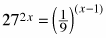3.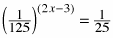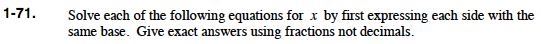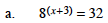$(2^3)^{(x+3)}=2^5$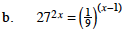$(3^3)^{2x}=(3^{-2})^{(x-1)}$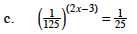$(5^{-3})^{(2x-3)}=5^{-2}$